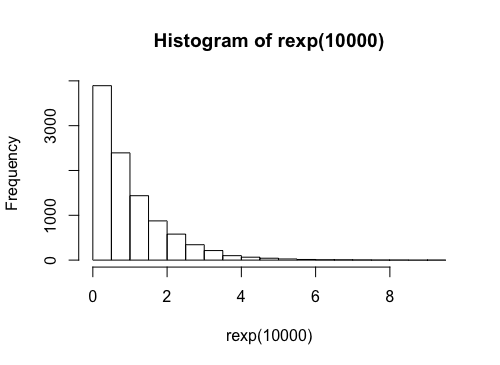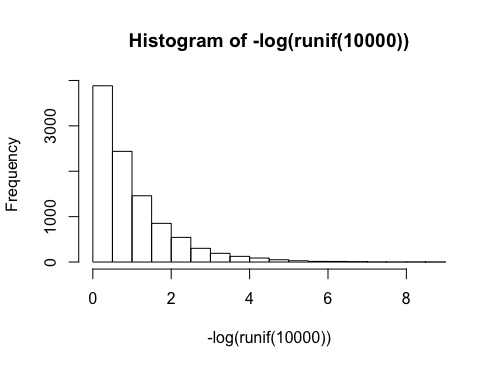# Metropolis-Hastings Sampling Tips

You may wonder why $\log f(x) - {\rm exprand}(1)$ is the same as $u \sim {\rm Uniform}(0, f(x))$.

% r\\ & \Leftrightarrow \log \min \left( 1, \frac{p(x|\theta^{'})}{p(x|\theta)} \right) > \log r\\ & \Leftrightarrow \log \min \left( 1, \frac{p(x|\theta^{'})}{p(x|\theta)} \right) - \log r > 0\\ & \Leftrightarrow \log \min \left( 1, \frac{p(x|\theta^{'})}{p(x|\theta)} \right) + {\rm exprand}(1) > 0, \end{align} %]]>

because

%$u \sim {\rm Uniform}(0, f(x))$ is the same as
%
%